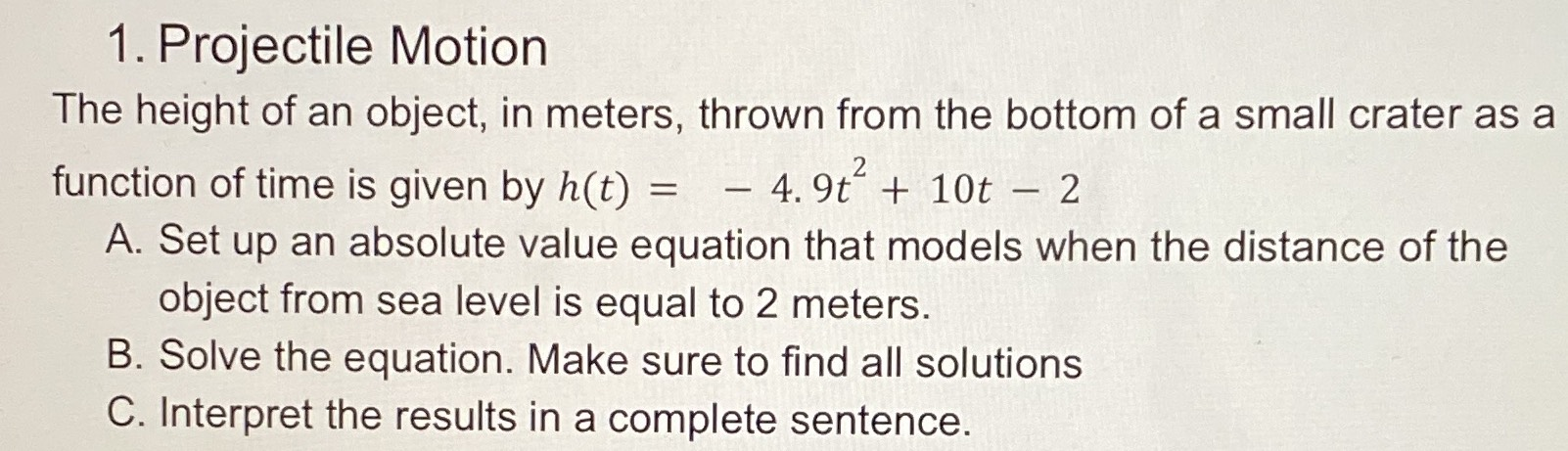### ¿Todavía tienes preguntas de matemáticas?

Pregunte a nuestros tutores expertos
Algebra
Pregunta1. Projectile Motion The height of an object, in meters, thrown from the bottom of a small crater as a function of time is given by $$h ( t ) = - 4.9 t ^ { 2 } + 10 t - 2$$

A. Set up an absolute value equation that models when the distance of the object from sea level is equal to $$2$$ meters. B. Solve the equation. Make sure to find all solutions C. Interpret the results in a complete sentence.

$$- 4.9 t ^ { 2 } + 10 t - 2 = 2$$
$$4.9 t ^ { 2 } - 10 t + 4 = 0$$
$$t = \frac { 10 \pm \sqrt { 100 - 16 \times 4.9 } } { 9.8 }$$
$$= \frac { 10 \pm 4.65 } { 9.8 } \approx 1.49$$ or $$0.55$$